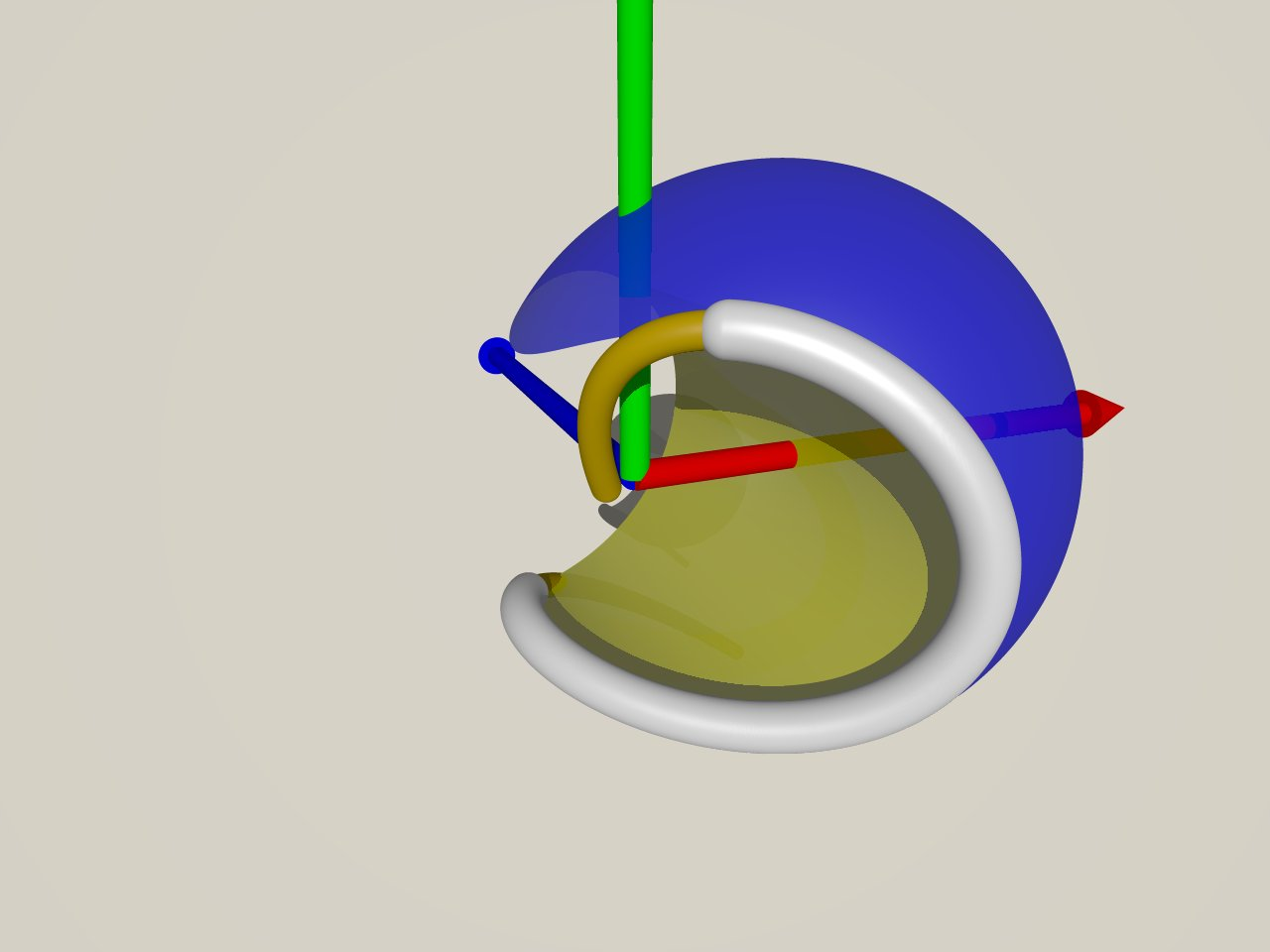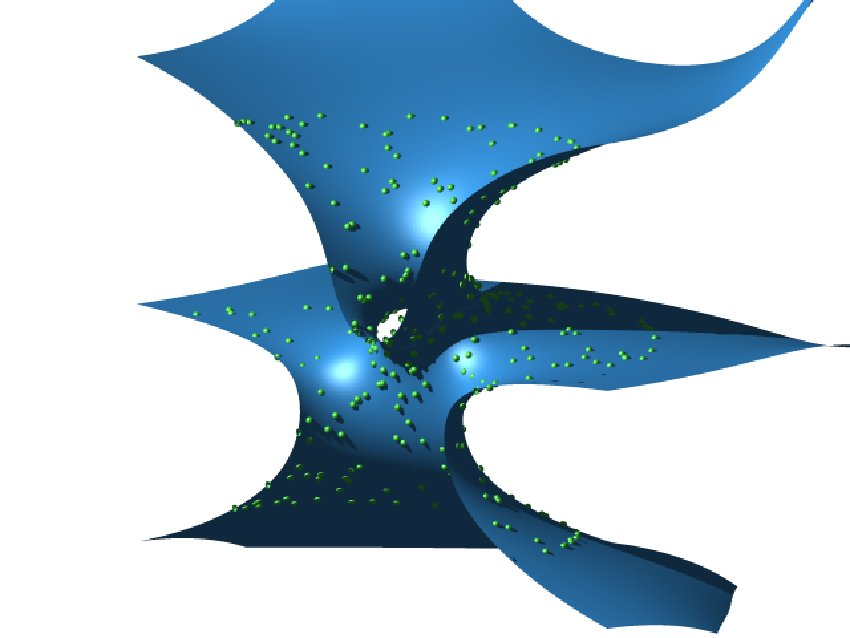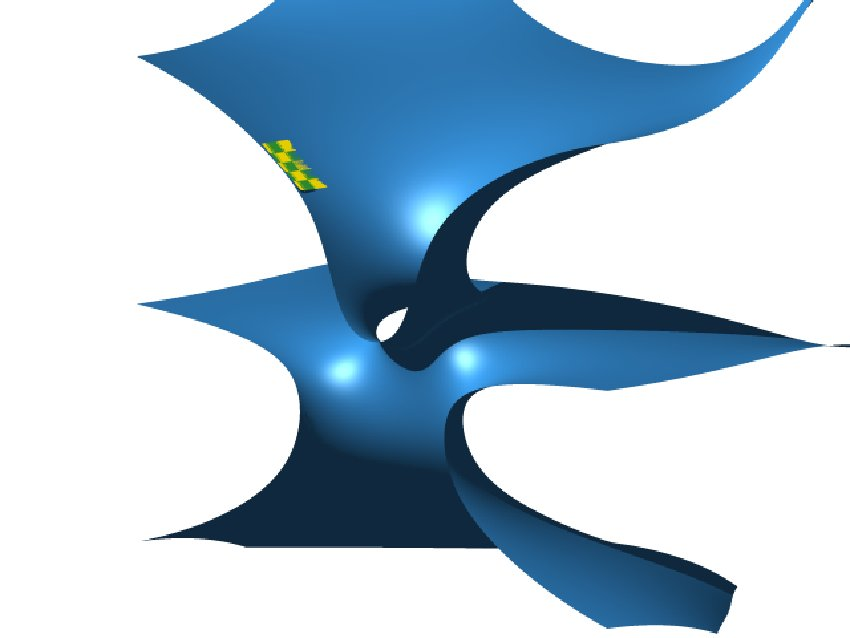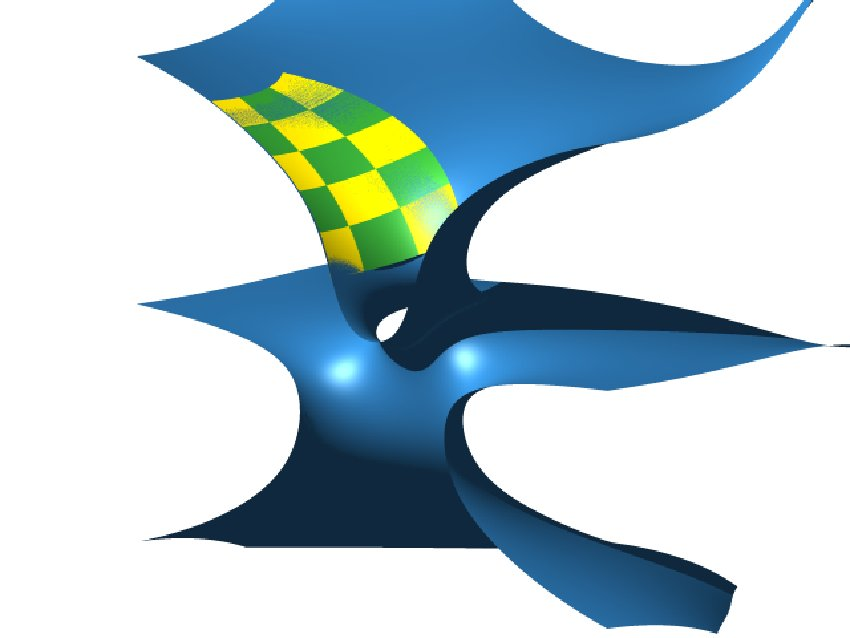Approximate parameterization
In the case of algebraic curves and surfaces, various symbolic  computation based techniques exist. However, these techniques have difficulties if the implicitly defined curve or surface is only given by floating point coefficients, i.e., with limited accuracy. Also, they cannot deal with general algebraic curves and surfaces, since an exact rational parameterization does not exist in the generic case. Approximate techniques, which generate a parameterization within a certain region of interest, can be used to avoid these problems. We discuss approximate techniques for parameterizing planar curves, space curves (given as the intersection of two surfaces), and surfaces.

## Planar curves

We developed a method for approximate parameterization of a planar algebraic curve by a rational Bezier (spline) curve. Our approach is based on the minimization of a suitable linear objective function, which takes both the distance from the curve and the positivity of the weight function (i.e., the numerator of the rational parametric representation) into account. The solution is computed by using an SQP type optimization technique. In addition, we use a region growing type approach in order to obtain a good initial solution, which is crucial for the convergence of the nonlinear optimization procedure. ¨

## Space curves

Algebraic space curves, which can be defined as the intersection of two algebraic surfaces, arise in various ways in geometric modeling, e.g., as the intersections of two natural quadrics; Some algebraic space curves, such as truly spatial cubics, admit an exact representation as rational parametric curves, and various parameterization techniques are known from classical algebraic geometry. More often, however, such a representation does not exist. In the general case, no such results can be expected, and the use of approximate techniques is unavoidable. Ideally, approximate techniques would be able to reproduce exact rational parameterizations, if those are available.

We developed a method for computing a rational curve which  approximates the intersection curves of two implicitly defined surfaces. Based on a preconditioning of the two given surfaces, the  problem can be formulated as an optimization problem, where the  objective function approximates the integral of the squared  Euclidean distance of the curve to the intersection curve. An SQP-type method is used to solve the optimization problem numerically. Special attention is paid to the generation of an initial solution, which is found by a region--growing--type technique. We briefly discuss how to treat singularities of the  intersection curve.

## Surfaces

An approximate parametrization method for surfaces is developed by the project partner Johannes Kepler University Linz. The method based on approximate implicitization method, an implicit surface which fit the given sample points is computed. Then, the algebraic surface is approximated by rational patches.

The figure below shows the parameterization of a sample of 6752 points, acquired from a minimal surface from the Costa-Hoffman-Meeks surface family. The genus of the surface is 1. An exact parameterization does not exist. We first compute an algebraic approximation. Based on the piecewise implicit description we find finite rational patches that approximate the given point cloud. Note that figure shows only half of the symmetric surface. The other half has been cut away for better visibility.

Published June 7, 2005Example 1. Two quadrics  intersect in  an  algebraic curve  of order  4. We  have approximated  it using  a  rational cubic curve. The thick white part of  the curve is the image of the interval [0,1].Example 2A. 6752 points, acquired from a minimal surface from the Costa-Hoffman-Meeks surface family are approximated by an piecewise algebraic surface.Example 2B.  We fit a finite rational patches that approximate the given point cloudExample 2C. The rational patch is gradually grown to cover more of the surface.